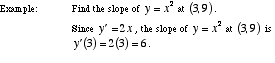# slope of a curve

Slope of a Curve

A number which is used to indicate the steepness of a curve at a particular point. The slope of a curve at a point is defined to be the slope of the tangent line. Thus the slope of a curve at a point is found using the derivative.